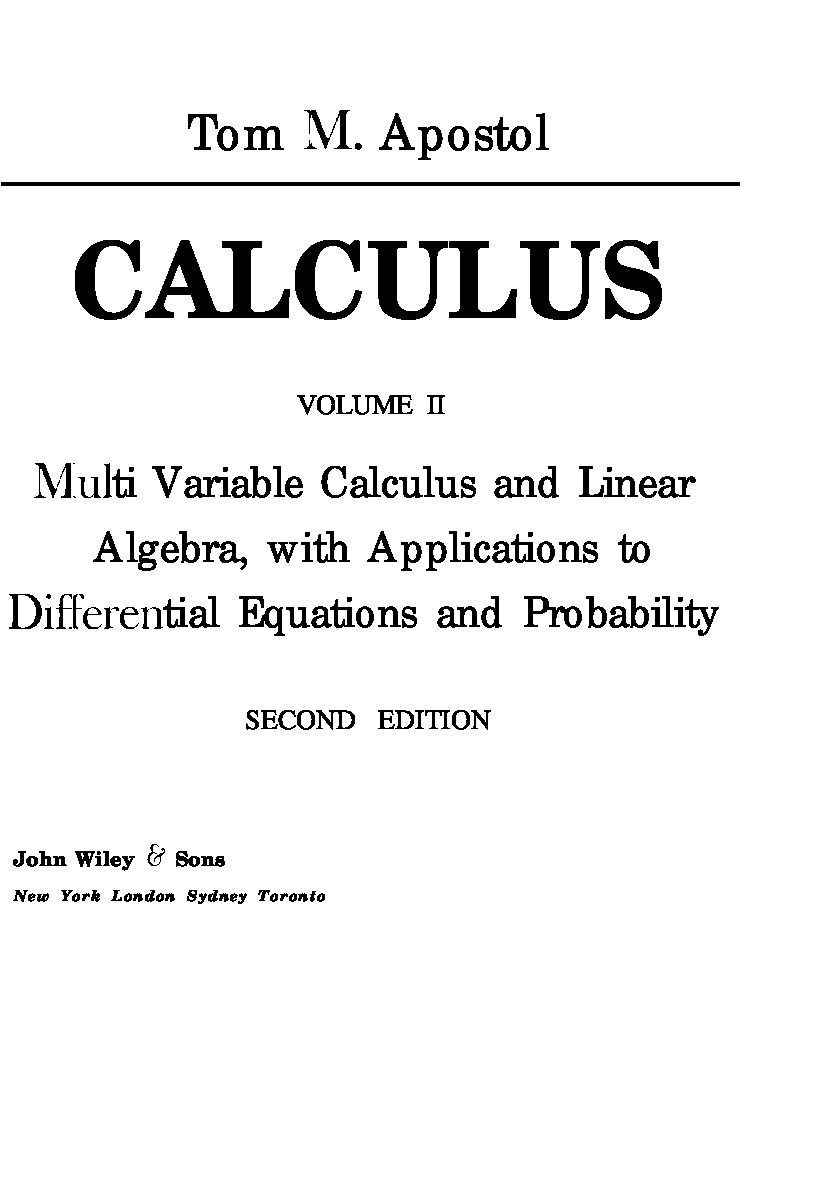# Apostol – CALCULUS – VOLUME 2 – Multi Variable Calculus and Linear Algebra, with Applications to Differential Equations and ProbabilityApostol-CALCULUS-VOLUME-2-Multi-Variable-Calculus-and-Linear-Algebra-with-Applications-to-Differential-Equations-and-Probability.pdf